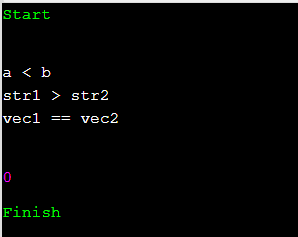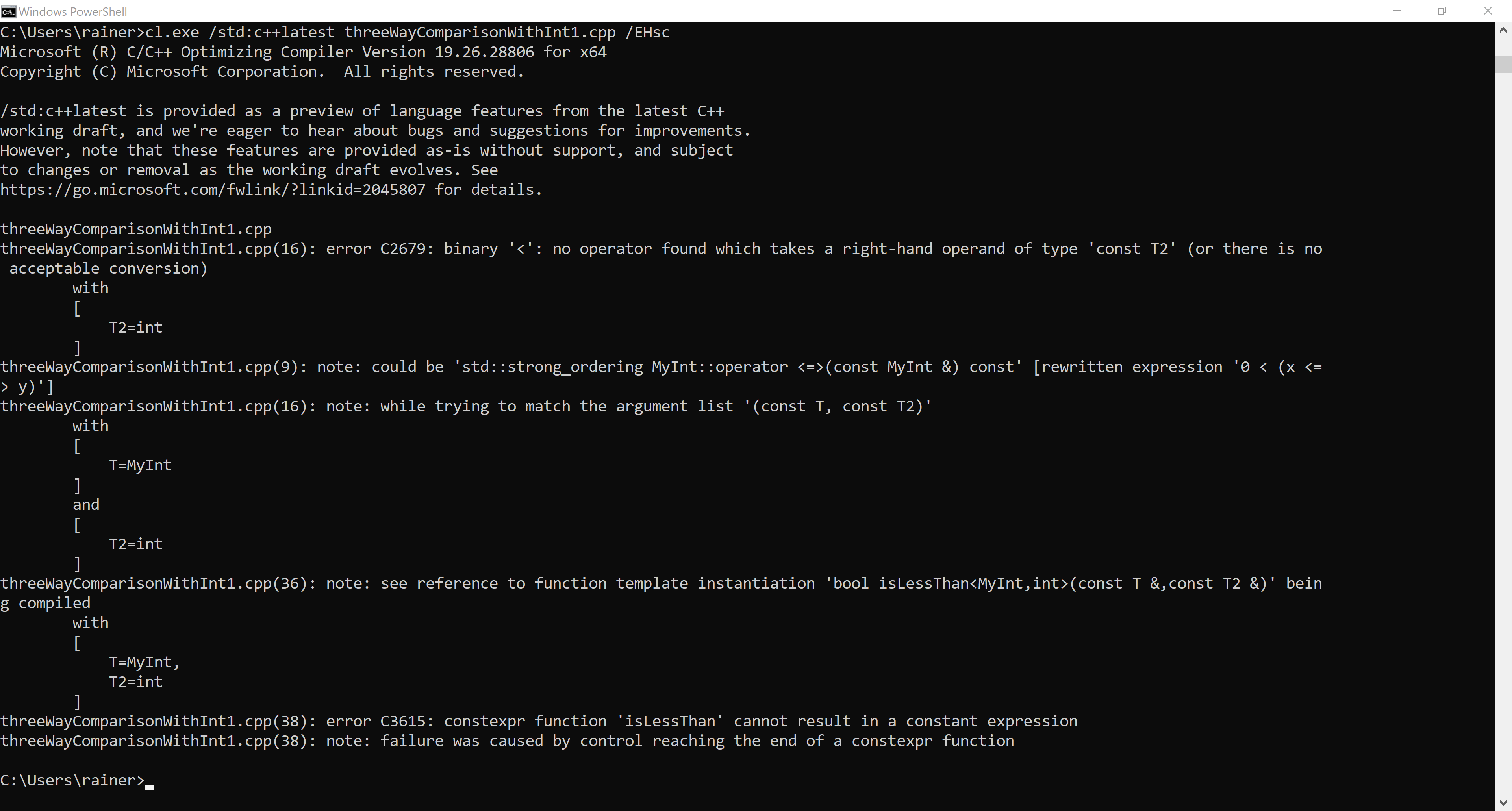# C++20: More Details to the Spaceship Operator

The compiler performs cleverly when it generates all six comparison operators. Ultimately, you get intuitive and efficient comparison operators for free. Let me dive into this post into the details of the spaceship operator.First, I want to add something I should have written about in my first post to the three-way comparison operator: “C++20: The Three-Way Comparision Operator“.

## Direct Usage of the Three-Way Comparison Operator

You can directly use the spaceship operator:

```// spaceship.cpp

#include <compare>
#include <iostream>
#include <string>
#include <vector>

int main() {

std::cout << std::endl;

int a(2011);
int b(2014);
auto res = a <=> b;                 // (1)
if (res < 0) std::cout << "a < b" << std::endl;
else if (res == 0) std::cout << "a == b" << std::endl;
else if (res > 0) std::cout << "a > b" << std::endl;

std::string str1("2014");
std::string str2("2011");
auto res2 = str1 <=> str2;          // (2)
if (res2 < 0) std::cout << "str1 < str2" << std::endl;
else if (res2 == 0) std::cout << "str1 == str2" << std::endl;
else if (res2 > 0) std::cout << "str1 > str2" << std::endl;

std::vector<int> vec1{1, 2, 3};
std::vector<int> vec2{1, 2, 3};
auto res3 = vec1 <=> vec2;          // (3)
if (res3 < 0) std::cout << "vec1 < vec2" << std::endl;
else if (res3 == 0) std::cout << "vec1 == vec2" << std::endl;
else if (res3 > 0) std::cout << "vec1 > vec2" << std::endl;

std::cout << std::endl;

}
```

##Modernes C++ Mentoring

Be part of my mentoring programs:

• "Fundamentals for C++ Professionals" (open)
• "Design Patterns and Architectural Patterns with C++" (open)
• "C++20: Get the Details" (reopens December 2023)
• Do you want to stay informed about my mentoring programs: Subscribe via E-Mail.

You can directly use the spaceship operator for int‘s (1), string‘s (2), and vector’s (3). Thanks to the wandbox online-compiler and the newest GCC, here is the program’s output.Now, it’s time for something new in C++. C++20 introduces the concept of “rewritten” expressions.

## Rewriting Expressions

When the compiler sees something such as a < b, it rewrites it to (a <=> b) < 0 using the spaceship operator.

Of course, the rule applies to all six comparison operators:

a OP b becomes (a <=> b) OP 0. It’s even better. If there is no conversion of the type(a) to the type(b), the compiler generates the new expression 0 OP (b <=> a).

For example, this means for the less-than operator, if (a <=> b) < 0 does not work, the compiler generates 0 < (b <=> a). In essence, the compiler automatically takes care of the symmetry of the comparison operators.

Here are a few examples of the rewriting expressions:

```// rewrittenExpressions.cpp

#include <compare>
#include <iostream>

class MyInt {
public:
constexpr MyInt(int val): value{val} { }
auto operator<=>(const MyInt& rhs) const = default;
private:
int value;
};

int main() {

std::cout << std::endl;

constexpr MyInt myInt2011(2011);
constexpr MyInt myInt2014(2014);

constexpr int int2011(2011);
constexpr int int2014(2014);

if (myInt2011 < myInt2014) std::cout << "myInt2011 < myInt2014" << std::endl;          // (1)
if ((myInt2011 <=> myInt2014) < 0) std::cout << "myInt2011 < myInt2014" << std::endl;

std::cout << std::endl;

if (myInt2011 < int2014) std:: cout << "myInt2011 < int2014" << std::endl;             // (2)
if ((myInt2011 <=> int2014) < 0) std:: cout << "myInt2011 < int2014" << std::endl;

std::cout << std::endl;

if (int2011 < myInt2014) std::cout << "int2011 < myInt2014" << std::endl;              // (3)
if (0 < (myInt2014 <=> int2011)) std:: cout << "int2011 < myInt2014" << std::endl;     // (4)

std::cout << std::endl;

}

```

I used in (1), (2), and (3) the less-than operator and the corresponding spaceship expression. (4) is the most exciting example. It exemplifies how the comparison (int2011 < myInt2014) triggers the generation of the spaceship expression (0 < (myInt2014 <=> int2011).

MyInt has an issue.  Constructors taking one argument should be explicit.

## Explicit Constructor

Constructors taking one argument, such as MyInt(int val) are conversion constructors. In the concrete case, an instance from MyInt can be generated from any integral or floating-point value because each integral or floating-point value can implicitly be converted to int. I assume you don’t want implicit conversion from an integral or a floating-point value when an instance of MyInt is required.

### First Try

To disable this implicit conversion, I make the constructor explicit following the Python meta-rule: explicit is better than implicit. The following program shows the explicit constructor:

```// threeWayComparisonWithInt1.cpp

#include <compare>
#include <iostream>

class MyInt {
public:
constexpr explicit MyInt(int val): value{val} { }
auto operator<=>(const MyInt& rhs) const = default;
private:
int value;
};

template <typename T, typename T2>
constexpr bool isLessThan(const T& lhs, const T2& rhs) {
return lhs < rhs;                                          // (1)
}

int main() {

std::cout << std::boolalpha << std::endl;

constexpr MyInt myInt2011(2011);
constexpr MyInt myInt2014(2014);

constexpr int int2011(2011);
constexpr int int2014(2014);

std::cout << "isLessThan(myInt2011, myInt2014): "
<< isLessThan(myInt2011, myInt2014) << std::endl;

std::cout << "isLessThan(int2011, myInt2014): "
<< isLessThan(int2011, myInt2014) << std::endl;  // (3)

std::cout << "isLessThan(myInt2011, int2014): "
<< isLessThan(myInt2011, int2014) << std::endl;  // (2)

constexpr auto res = isLessThan(myInt2011, int2014);

std::cout << std::endl;

}
```

This was easy. Thanks to the explicit constructor, the implicit conversion from int to MyInt in (1) is not valid anymore. The compiler now speaks an unambiguous message.When you read the error message carefully, you notice that there is no operator < for a right-hand operand int available and no conversion from int to MyInt possible. Interestingly, the compiler complains about (2) but not about (3). Both function calls cause a compiler error.

### Second Try

To support the comparison from MyInt‘s and int‘s, MyInt needs an additional three-way comparison operator.

```#include <compare>
#include <iostream>

class MyInt {
public:
constexpr explicit MyInt(int val): value{val} { }
auto operator<=>(const MyInt& rhs) const = default; // (4)
constexpr auto operator<=>(const int& rhs) const {  // (1)
return value <=> rhs;
}
private:
int value;
};

template <typename T, typename T2>
constexpr bool isLessThan(const T& lhs, const T2& rhs) {
return lhs < rhs;
}

int main() {

std::cout << std::boolalpha << std::endl;

constexpr MyInt myInt2011(2011);
constexpr MyInt myInt2014(2014);

constexpr int int2011(2011);
constexpr int int2014(2014);

std::cout << "isLessThan(myInt2011, myInt2014): "
<< isLessThan(myInt2011, myInt2014) << std::endl; // (3)

std::cout << "isLessThan(int2011, myInt2014): "
<< isLessThan(int2011, myInt2014) << std::endl;   // (3)

std::cout << "isLessThan(myInt2011, int2014): "
<< isLessThan(myInt2011, int2014) << std::endl;   // (3)

constexpr auto res = isLessThan(myInt2011, int2014);        // (2)

std::cout << std::endl;

}
```

I defined in (1) the three-way comparison operator and declared it constexpr. The user-defined three-way comparison operator is in contrast to the compiler-generated three-way comparison operator, not constexpr. Consequently, I can perform the isLessThan (4) call at compile-time. Comparing MyInt’s and int’s is possible in each combination (3).I find the implementation of the various three-way comparison operators very elegant. The compiler auto-generates the comparison of MyInt’s, and the user defines the comparison with int‘s explicitly.  Additionally, you have to define only two operators to get 18 = 3 * 6 combinations of comparison operators. 3 represents the combination of int‘s and MyInt‘s and 6 for the six comparison operators. I discussed in my last post, “C++20: The Three-Way Comparision Operator”  the 18 operators you had to overload before C++20.

I want to make one point clear: You can even compare MyInt which each type that is convertible to int.

Stop! You may ask yourself: What is the current implementation using an explicit constructor

```class MyInt {
public:
constexpr explicit MyInt(int val): value{val} { }
auto operator<=>(const MyInt& rhs) const = default;
constexpr auto operator<=>(const int& rhs) const {
return value <=> rhs;
}
private:
int value;
};
```

better than the previous implementation using a constructor capable of implicit conversions? Both classes allow comparisons with integrals and floating-point values.

```class MyInt {
public:
constexpr MyInt(int val): value{val} { }
auto operator<=>(const MyInt& rhs) const = default;
private:
int value;
};W
```

## What’s next?

There is a subtle difference between an explicit and a non-explicit constructor for MyInt that you can easily see when I make MyInt more int-like in my next post. Additionally, the compiler-generated == and != operators are special for performance reasons, and the interplay of classical comparison operators and the three-way comparison operator are worth an extra post.

Thanks a lot to my Patreon Supporters: Matt Braun, Roman Postanciuc, Tobias Zindl, G Prvulovic, Reinhold Dröge, Abernitzke, Frank Grimm, Sakib, Broeserl, António Pina, Sergey Agafyin, Андрей Бурмистров, Jake, GS, Lawton Shoemake, Jozo Leko, John Breland, Venkat Nandam, Jose Francisco, Douglas Tinkham, Kuchlong Kuchlong, Robert Blanch, Truels Wissneth, Kris Kafka, Mario Luoni, Friedrich Huber, lennonli, Pramod Tikare Muralidhara, Peter Ware, Daniel Hufschläger, Alessandro Pezzato, Bob Perry, Satish Vangipuram, Andi Ireland, Richard Ohnemus, Michael Dunsky, Leo Goodstadt, John Wiederhirn, Yacob Cohen-Arazi, Florian Tischler, Robin Furness, Michael Young, Holger Detering, Bernd Mühlhaus, Matthieu Bolt, Stephen Kelley, Kyle Dean, Tusar Palauri, Dmitry Farberov, Juan Dent, George Liao, Daniel Ceperley, Jon T Hess, Stephen Totten, Wolfgang Fütterer, Matthias Grün, Phillip Diekmann, Ben Atakora, Ann Shatoff, Rob North, Bhavith C Achar, and Marco Parri Empoli.

Thanks, in particular, to Jon Hess, Lakshman, Christian Wittenhorst, Sherhy Pyton, Dendi Suhubdy, Sudhakar Belagurusamy, Richard Sargeant, Rusty Fleming, John Nebel, Mipko, Alicja Kaminska, Slavko Radman, and David Poole.

 My special thanks to EmbarcaderoMy special thanks to PVS-StudioMy special thanks to Tipi.buildMy special thanks to Take Up Code## Seminars

I’m happy to give online seminars or face-to-face seminars worldwide. Please call me if you have any questions.

### Standard Seminars (English/German)

Here is a compilation of my standard seminars. These seminars are only meant to give you a first orientation.

• C++ – The Core Language
• C++ – The Standard Library
• C++ – Compact
• C++11 and C++14
• Concurrency with Modern C++
• Design Pattern and Architectural Pattern with C++
• Embedded Programming with Modern C++
• Generic Programming (Templates) with C++

#### New

• Clean Code with Modern C++
• C++20

### Contact Me

Modernes C++ Mentoring,Tags:
0 replies